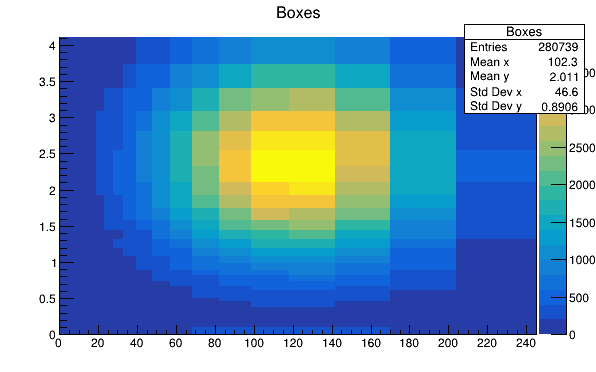# Th 2Poly Boxes¶

This tutorial illustrates how to create an histogram with polygonal bins (TH2Poly). The bins are boxes.

Author: Olivier Couet
This notebook tutorial was automatically generated with ROOTBOOK-izer from the macro found in the ROOT repository on Tuesday, May 24, 2022 at 04:00 PM.

In :
TCanvas *ch2p2 = new TCanvas("ch2p2","ch2p2",600,400);
gStyle->SetPalette(57);
TH2Poly *h2p = new TH2Poly();
h2p->SetName("Boxes");
h2p->SetTitle("Boxes");

Int_t i,j;
Int_t nx = 40;
Int_t ny = 40;
Double_t xval1,yval1,xval2,yval2;
Double_t dx=0.2, dy=0.1;
xval1 = 0.;
xval2 = dx;

for (i = 0; i<nx; i++) {
yval1 = 0.;
yval2 = dy;
for (j = 0; j<ny; j++) {
yval1 = yval2;
yval2 = yval2+yval2*dy;
}
xval1 = xval2;
xval2 = xval2+xval2*dx;
}

TRandom ran;
for (i = 0; i<300000; i++) {
h2p->Fill(50*ran.Gaus(2.,1), ran.Gaus(2.,1));
}

h2p->Draw("COLZ");
return ch2p2;


Draw all canvases

In :
gROOT->GetListOfCanvases()->Draw()